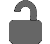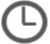• Register

The only FREE source for complete Lean Six Sigma training!

## Variation Over Time (Short/Long Term Data)

A review of short and long term data and the impacts that variation has over time.

•Mar 15, 2018 posted by Matt Hansen

Thanks John. I don't know the full history behind the situation, but from what I've heard, the 1.5 sigma issue was something that was derived from a situation or project many years ago (perhaps at Motorola or GE), but the debate is centered around there not being much evidence to support it. It's generally used as an estimate, so instead, I recommend just doing some process capability analysis that calculates the short/long term values for you (also expressed as Cpk vs. Ppk), then you won't have to worry about it. Frankly, in my many years of using LSS across hundreds of projects, I may have only encountered the potential use of that 1.5 sigma factor a couple of times.

•Mar 11, 2018 posted by John Maxwell

Hi Matt. In the textbook there is a note about debate over the validity of 1.5sigma as an accepted variation for short-term processes over time. Would you expound on the debate? Thanks. John Maxwell

 Below are 36 videos (less than 10 hours of content) selected from the 121 videos in the Advanced Training Path. These videos go beyond the basics of Lean Six Sigma to help you apply the tools & concepts, but without getting as complex or detailed as the certification level in the Advanced Training Path. 1. StatStuff Orientation (What is StatStuff?)This video welcomes you to StatStuff by explaining some general concepts about how the videos are designed and how the site works. 2. Introduction to Lean and Six SigmaAn introduction to the fundamental concepts of the Lean and Six Sigma methodologies using the IPO model. 3. Lean and Six Sigma Project MethodologiesAn introduction to five project methodologies (Lean, DMAIC, DMADV, DFSS & PMI) and when to use each. 4. Corporate CTQ DrilldownA review of how to align a project opportunity to the overall business strategy and needs by understanding the business CTQs in a CTQ Drilldown. 5. Project Financial BenefitsA review of how to identify and categorize financial benefits from a project. 6. Prioritization Using a QFD ToolA review of how a Quality Functional Deployment (QFD) tool can be used to prioritize items, such as project opportunities. 7. Project Pre-Assessment Using a Min/Max AnalysisA review of why it’s important to do a project pre-assessment and how to do it using a Min/Max Analysis. 8. Key Roles in a Lean or Six Sigma ProjectA review of the project and functional roles in Lean Six Sigma projects like Green Belt, Black Belt, Sponsor, Champion, etc. 9. Developing a Project Strategy Using IPO-FAT ToolA review of how to build a strategy for a project and how the IPO-FAT tool can be used for developing that strategy. 10. Introduction to LeanAn introduction to Lean including a brief history, the philosophy of Lean, and a summary of some common Lean tools and concepts. 11. Problem Resolution Using DMAICA review of how the DMAIC methodology follows the typical steps we follow when trying to resolve a problem. 12. The DMAIC Roadmap (Levels 1 & 2)A high-level roadmap through the DMAIC methodology that navigates the user through the various tools and concepts for leading a Six Sigma project. 13. Adapting Lean to Six Sigma DMAIC FlowA description of how the Lean tools and concepts can be adapted to the Six Sigma DMAIC methodology. 14. Define Phase Roadmap (Level 3)A detailed roadmap through the Define phase of the DMAIC methodology that navigates the user through the various tools and concepts for leading a Six Sigma project. 15. Measure Phase Roadmap (Level 3)A detailed roadmap through the Measure phase of the DMAIC methodology that navigates the user through the various tools and concepts for leading a Six Sigma project. 16. Analyze Phase Roadmap (Level 3)A detailed roadmap through the Analyze phase of the DMAIC methodology that navigates the user through the various tools and concepts for leading a Six Sigma project. 17. Improve Phase Roadmap (Level 3)A detailed roadmap through the Improve phase of the DMAIC methodology that navigates the user through the various tools and concepts for leading a Six Sigma project. 18. Control Phase Roadmap (Level 3)A detailed roadmap through the Control phase of the DMAIC methodology that navigates the user through the various tools and concepts for leading a Six Sigma project. 19. Risk Analysis: The Reason We Use StatisticsA review of the importance of risk in our decision-making and how statistics can be used to measure that risk. 20. Analysis of Behavior & Cognition (ABC) ModelA review of the ABC model that explores how we think so we can understand the risks and evidence behind our decisions and how to influence others. 21. Transfer FunctionA review of the transfer function and the critical part it plays as a fundamental concept in the DMAIC methodology. 22. Hypothesis Testing: OverviewAn introductory overview to an extended series about hypothesis testing. This lesson includes the general 4 step process used for hypothesis testing. 23. Overview of Statistical Terms and ConceptsA high-level review of the fundamental terms and concepts associated with statistics, such as population vs. sample data, distributions, etc. 24. Building a Problem StatementA review of what a problem statement and background statement are and the characteristics of an ideal problem statement. 25. Defining the Project YA review of why we need to define a project Y and some methods for ensuring we’re defining the right project Y. 26. Identify Root Causes: C&E DiagramAn extension of the topic on identifying root causes using a cause & effect (C&E) diagram that will lead toward building a data collection plan (DCP). 27. Identify Root Causes: 5 WhysAn extension of the topic on identifying root causes using a 5 Whys approach that will lead toward building a data collection plan (DCP). 28. Identify Root Causes: C&E MatrixAn extension of the topic on identifying root causes by showing how the C&E Matrix is used after a C&E diagram and 5 Whys for building a DCP. 29. MSA: OverviewThe first of an extended series on conducting a measurement system analysis (MSA) to help test the reliability of collected data. 30. Calculating a Sample SizeA review of how to calculate a sample size using a Sample Size Calculator. 31. Variation Over Time (Short/Long Term Data)A review of short and long term data and the impacts that variation has over time. 32. Hypothesis Testing: Finding the Right Statistical TestAn extension on a series about hypothesis testing, this lesson reviews a chart that can help you find the right statistical test for your analysis. 33. Hypothesis Testing: Statistical Laws and Confidence IntervalsAn extension on a series about hypothesis testing, this lesson introduces some statistical concepts that are fundamental to most hypothesis testing. 34. Hypothesis Testing: Proportions (Compare 1:1)An extension on a series about hypothesis testing, this lesson reviews the 2 Proportions Test as a measurement of proportions. 35. Hypothesis Testing: Relationships (Overview)An extension on hypothesis testing, this lesson introduces the concepts of a correlation and regression as part of measuring statistical relationships. 36. Hypothesis Testing: Relationships (Compare 1:1)An extension on hypothesis testing, this lesson reviews the Pearson Correlation and Fitted Line Plot as part of measuring statistical relationships.